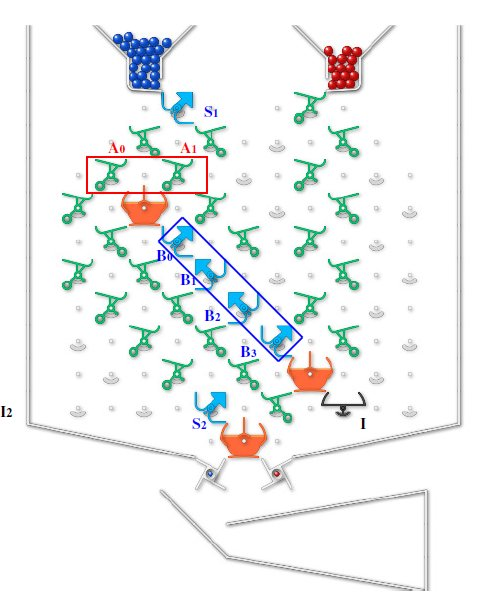# Integer division (4-bits divided by 2-bits)

Hello,
here we propose a new challenge (Integer division) which is a dual problem of challenge 56.
The dividend is set in the register B (4-bits up to 15 decimal value) and the divisor is set and fixed in register A (2-bits up to 3 as decimal value).
The scheme is the dual of the multiplication and it consists in a sequence of subtractions of A from B (result updating in B).
The result of the integer division is the number of red balls which are collected in the output chain.
Interceptor I determines the end of the process, when the register B passes through zero (overflow)
So far we have been unable to estimate the value of the reminder of such integer division
(there are differences between even and odd values of the divisor register A).
Division by zero should be not-permitted; anyway, if A is set to 0, the process will never come to end and the output chain of red-balls will continue indefinitely.
The example in the attached picture is for the division 9/3.Starting-conditions: Service bits: S1=1 S2=1; starting-ball: blue
Looking forward to any comment,
Bye
Leonardo & Gio